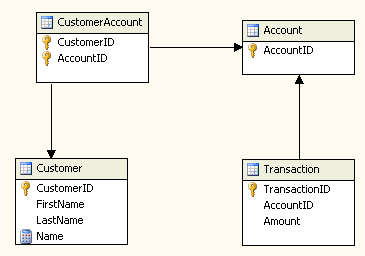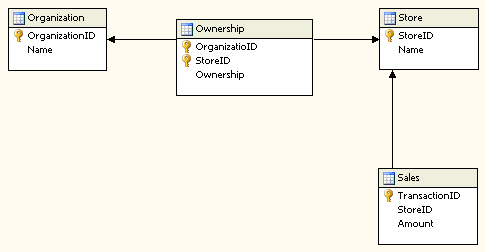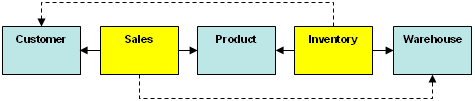# Many-to-Many Dimensions in Analysis Services 2005

Richard Tkachuk
Microsoft Corporation

June 2005

Applies to:

Microsoft SQL Server 2005 Analysis Services

Summary: See an example of using the Many-to-Many dimension in SQL Server 2005 Analysis Services to analyze sales data, and get ideas for other uses such as treating medical conditions, software testing, and more. (8 printed pages)

#### Contents

Introduction
Defining a Many-to-Many Dimension in Analysis Services 2005
Aggregating Data in Many-to-Many Dimensions
Measure Expressions and Many-to-Many Dimensions
Chaining Many-to-Many Dimensions
Resolving Recursion: Many-to-Many Dimensions
Many-to-Many Dimensions and Calculations
Conclusion

## Introduction

Data structures do not always conform to the snowflake or star schema model where one fact is associated with a single dimension member. For example, in a typical cube analyzing sales data, a single sales transaction is associated with a single customer, a single product, and a single point in time.

Data structures can be more complex. For example, consider the example of financial transactions in accounts that can have one or more customers. This can be modeled as:The relationship between transaction and customer is a many-to-many relationship. A single transaction can be associated with many customers and each customer can be associated with many transactions. Say we have 3 customers, John and Jane Hanover, who share a joint account, and Henry Waxman, who has an individual account. If John and Jane contribute \$100.00 to their account and Henry contributes \$150.00, the results for all customers looks like this:The sum for all amounts is not the sum of the individual amounts for each customer—that would double-count the data that John and Jane share. Instead, the total amount is the sum of all transactions.

When computing a balance for a customer, the amount is the aggregate of all transactions to the accounts that customer is associated with, and not the simple sum of the transactions for each customer.

## Defining a Many-to-Many Dimension in Analysis Services 2005

In the example above, there are two dimensions and two measure groups. The Customer dimension is the Many-to-Many (MM) dimension to the Transaction measure group. The Customer Account measure group is the Intermediate Measure Group and Account is the intermediate dimension. The intermediate measure group is the measure group that relates the Many-to-Many dimension to the regular dimension. In this case, it relates the Customer dimension to Account dimension. The transaction measure group is related to Customer in the conventional manner, but is also related to the Customer dimension via a many-to-many relationship. In the Dimension Usage editor, it looks like this:What this means is that the amount for each customer is the aggregate amount for each account the customer belongs to.

## Aggregating Data in Many-to-Many Dimensions

As shown in the previous example, the amount for each member in the customer dimension is the aggregate for each account the customer is associated with. Except for the all member of the MM dimension, this rule applies to all the attributes in the MM dimension:

Except for the all member, the value for each member in a many-to-many dimension is the aggregate of the distinct set of granularity attribute members that exist with the many-to-many dimension member across the intermediate measure group.

This is a mouthful that might be best explained with an example. Include the attribute gender in the customer dimension and browse the data by gender. The results look like this:The amount for females is the amount for the single female in our database. And the amount for males is the value for both John Hanover and Henry Waxman.

The all member is somewhat special. Instead of aggregating the values of the members of the key attribute that exist with it, it is the simple aggregate of all values in the fact table. In other words:

The amount for the all member of a Many-to-Many dimension is the aggregate of all facts.

So even if the table sourcing the intermediate measure group (CustomerAccount, in this case) is empty, the result will be unchanged.

## Measure Expressions and Many-to-Many Dimensions

Values in a many-to-many dimension do not always aggregate as the distinct sum, but sometimes need to be multiplied by some factor. For example, consider the case of several organizations that share ownership in a chain of stores:If this model is populated with two stores and three organizations, the two stores have sales as follows:

 Store Sales Store 1 300 Store 2 700

And the three organizations share ownership of Store 1 and 2, as follows:

 Organization Store Ownership General Corporation Store 1 0.60 MegaStores Ltd Store 1 0.40 General Corporation Store 2 0.50 MegaStores Ltd Store 2 0.40 Dundee Corporation Store 2 0.10

Similar to the prior example, the Organization is a many-to-many dimension with respect to the Sales measure group. The difference is that the Amount measure in the Sales measure group has a Measure Expression defined:When computing the sales for each Organization, each Amount for each store is multiplied by the Ownership.

This instructs Analysis Services to multiply the amount for each store by the ownership of that store for row. The result is the weighted sales for each organization based on the percentage ownership:It is up to the cube author to ensure that the weightings are defined correctly; otherwise, values may be unexpected.

When a measure expression is defined, it may be expensive to compute the result for the all member of the Many-to-Many dimension if the product needs to be computed across an enormous number of rows. And in examples like this, the value for the all member is the simple sum of the fact table. There is one more property on a many-to-many dimension that accommodates this, the Direct Slice. This is a tuple on the Many-to-Many dimension, where the server is instructed to not compute the measure expression except where the cube author guarantees that the aggregate value of the facts matches the result of this expression. For example, in a scenario using measure expression for currency conversion where the sales facts are stored in US Dollars, Currency.USD would be the DirectSlice in the Currency dimension.

Note   Both Measure Expression and Direct Slice are not true MDX expressions. Measure expressions are constrained to be in the form of <measure 1> * <measure 2> or <measure 1> / <measure 2>. Direct slice should be a tuple defining a coordinate in each attribute hierarchy in the Many-to-Many dimension without any MDX function.

## Chaining Many-to-Many Dimensions

In more complex scenarios, many-to-many dimensions can be chained together with the restriction that the dimension must a dimension to all intervening measure groups.

Consider the diagram below:To add D1 as a many-to-many dimension to MG3, it must first be a many-to-many dimension to MG2 as well:In the diagram above, yellow represents fact tables and blue represents dimension tables. The dotted line represents a many-to-many dimension relationship.

## Resolving Recursion: Many-to-Many Dimensions

Many-to-many dimensions can be defined in a pretzel-like shape:When resolving the members in the MM dimension that exist with a measure group, other MM dimensions from the intermediate measure group are ignored. So when determining the Warehouses that exist with sales via the intermediate measure group Inventory, its other MM dimension, Customer, is ignored.

### Performance Considerations in Many-to-Many Dimensions

When members of a many-to-many dimension are included in a query, Analysis Services effectively performs a join between the Many-to-Many dimension and the regular dimension(s) across the intermediate measure group. Therefore, Many-to-Many dimension query performance is directly related to the number of rows in the intermediate measure group.

If performance is poor because of large number of rows in the intermediate measure group, consider denormalizing the MM dimension to reduce the number of rows.

## Many-to-Many Dimensions and Calculations

Calculations aggregate "up" in many-to-many dimensions like other dimensions. For example, if Customer.Geography is a many-to-many dimension and the cell (Customer.Geography.USA.WA) is populated with a calculation, it changes the value of its parent member to the simple sum of it and its siblings.

Visual totals apply to one level at a time only in many-to-many dimensions. For example, if Geography is a many-to-many dimension in the cube MMSales, then:

``````select measures.sales on columns,
VisualTotals({Geography.USA, Geography.USA.WA})
from MMSales
``````

returning the value of WA for USA, and

``````select measures.sales on columns,
VisualTotals({Geography.USA, Geography.USA.WA, Geogrpahy.USA.WA.Seattle })
from MMSales
``````

returns results of WA having the same value as Seattle, and USA will be the value of all of WA.

## Conclusion

Many-to-many dimensions are applicable in so many scenarios not discussed here, such as:

• Medical analyses where each condition can be treated with one or more medications
• Product sales where each product is composed of one or more items
• Software testing where each test addresses one or more scenarios

The list goes on and on—you can use many-to-many dimensions to extend analytics beyond the simpler star schema or snowflake model.